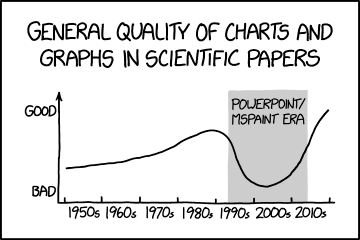# Mathematics

• Posted on: 12 December 2019
Block:
Term:
Year:
LA Code:
Code:
Room:
Learning Area:
Levels:
6

We are running three slightly different mathematics courses at level 6 (NCEA level 1) this year. ....

This course will aim to give students the best foundation for further study in maths and sciences. We will sit three out of the four externals.
The year plan is:

• Graphs 4 credits external
• Linear algebra 3 credits internal
• Algebra (MCAT) 4 credits external
• Chance and Data 4 credits external
• Elements of chance 3 credits internal
• Geometric Reasoning 4 credits external

To start with we will cover graphs, some of this this involves using devices so please make sure you have something for this class (it is possible on a phone, sometimes a little fiddly, try out desmos.com and see how functional you find it).

In Mathematics Graphs allow us to represent shapes with equations and visa vera. This allows us to link stuff in the 'real' world with mathematics. So for example you may graph something over time (velocity of a car, savings, debt, profit, radness..) and then make predictions about what will happen in the future.

• find optimal solutions, using numerical approaches
• solve linear equations and inequations, quadratic and simple exponential equations, and simultaneous equations with two unknowns
• relate graphs, tables, and equations to linear, quadratic, and simple exponential
• relationships found in number and spatial patterns relate rate of change to the gradient of a graph.Assessment Opportunities: